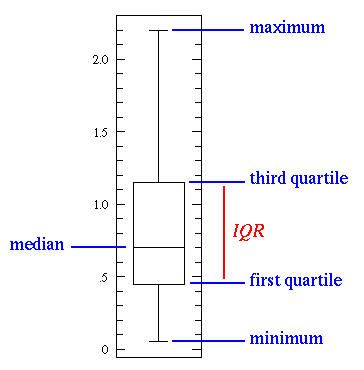# Some common Machine Learning, Statistics and Data Science terms starts with I

## I

[su_table]

### Word ### Description

Imputation Imputation is a technique used for handling missing values in the data. This is done either by statistical metrics like mean/mode imputation or by machine learning techniques like kNN imputation

For example,

If the data is as below

Name Age

Akshay 23
Akshat NA
Viraj 40

The second row contains a missing value, so to impute it we use mean of all ages, i.e.

Name Age

Akshay 23
Akshat 31.5
Viraj 40
Inferential Statistics In inferential statistics, we try to hypothesize about the population by only looking at a sample of it. For example, before releasing a drug in the market, internal tests are done to check if the drug is viable for release. But here we cannot check with the whole population for viability of the drug, so we do it on a sample which best represents the population.
IQR IQR (or interquartile range) is a measure of variability based on dividing the rank-ordered data set into four equal parts. It can be derived by Quartile3 – Quartile1.Iteration Iteration refers to the number of times an algorithm’s parameters are updated while training a model on a dataset. For example, each iteration of training a neural network takes certain number of training data and updates the weights by using gradient descent or some other weight update rule.